xThermal diffusivityEncyclopedia
In heat transfer
Heat transfer
Heat transfer is a discipline of thermal engineering that concerns the exchange of thermal energy from one physical system to another. Heat transfer is classified into various mechanisms, such as heat conduction, convection, thermal radiation, and phase-change transfer...

analysis, thermal diffusivity (usually denoted are also used) is the thermal conductivity
Thermal conductivity
In physics, thermal conductivity, k, is the property of a material's ability to conduct heat. It appears primarily in Fourier's Law for heat conduction....

divided by density
Density
The mass density or density of a material is defined as its mass per unit volume. The symbol most often used for density is ρ . In some cases , density is also defined as its weight per unit volume; although, this quantity is more properly called specific weight...

and specific heat capacity at constant pressure. It has the SI
Si
Si, si, or SI may refer to :- Measurement, mathematics and science :* International System of Units , the modern international standard version of the metric system...

unit of m²/s. The formula is: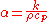where
•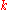is thermal conductivity (W/(m·K))
•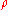is density (kg/m³)
•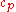is specific heat capacity (J/(kg·K))

The denominator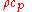can be considered the volumetric heat capacity
Volumetric heat capacity
Volumetric heat capacity , also termed volume-specific heat capacity, describes the ability of a given volume of a substance to store internal energy while undergoing a given temperature change, but without undergoing a phase change...

(J/(m³·K)).

In a sense, thermal diffusivity is the measure of thermal inertia.
In a substance with high thermal diffusivity, heat moves rapidly through because the substance conducts heat quickly relative to its volumetric heat capacity or 'thermal bulk'.

Thermal diffusivity is often measured with the flash method. It involves heating a cylindrical sample with a short energy pulse at one end and analyzing the temperature change at the other end.
Thermal diffusivity of selected materials and substances
Material Thermal diffusivity
(m²/s)
Thermal diffusivity
(mm²/s)
Pyrolytic graphite
Pyrolytic carbon
Pyrolytic carbon is a material similar to graphite, but with some covalent bonding between its graphene sheets as a result of imperfections in its production....

, parallel to layers
1.22 × 10−3 1220
Silver, pure (99.9%) 1.6563 × 10−4 165.63
Gold 1.27 × 10−4 127.
Copper 1.1234 × 10−4 112.34
Aluminium 8.418 × 10−5 84.18
Aluminum 6061-T6 Alloy 6.4 × 10−5 64.
Water vapour (1 atm, 400 K) 2.338 × 10−5 23.38
Air (1 atm, 300 K) 2.2160 × 10−5 22.16
Aluminium oxide (polycrystalline) 1.20 × 10−5 12.0
Steel, 1% carbon 1.172 × 10−5 11.72
Steel, stainless 304A 4.2 × 10−6 4.2
Iron 2.3 × 10−5 23.
Silicon 8.8 × 10−5 88
Quartz 1.4 × 10−6 1.4
Silicon Dioxide (Polycrystalline) 8.3 × 10−7 0.83
Water (300 K) 1.4 × 10−7 0.14
Polyvinyl Chloride (PVC) 8 × 10−8 0.08
Alcohol 7 × 10−8 0.07
Air 1.9 × 10−5 19
Argon (300 K, 1 atm) 22
Helium (300 K, 1 atm) 190
Hydrogen (300 K, 1 atm) 160
Nitrogen (300 K, 1 atm) 22
Pyrolytic graphite, normal to layers 3.6 × 10−6 3.6
Sandstone 1.12–1.19 × 10−6 1.15
Tin 4.0 × 10−5 40.
Brick, common 5.2 × 10−7 0.52
Glass, window 3.4 × 10−7 0.34
Rubber 1.3 × 10−7 0.13
Nylon 9 × 10−8 0.09
Wood (Yellow Pine) 8.2 × 10−8 0.082
Oil, engine (saturated liquid, 100 °C) 7.38 × 10−8 0.0738